## Designing timber compaction piles to achieve a target soil density using CPTu data

 Author(s) J Philpot, N. Barounis Published N/A Collection NZGS Symposium Link N/A Compilation 21st NZGS Symposium
Designing timber compaction piles to achieve a target soil density using CPTu data

ABSTRACT

For sites with shallow soil layers consisting of loose sand and/or potentially liquefiable soil, timber compaction piles (TCP) can be driven into the ground to improve the geotechnical performance of the site, particularly for land supporting residential and lightweight commercial or industrial buildings. The TCP are driven into the ground to increase the density and stiffness of shallow soil layers; however, there are limited design methods available for determining an efficient and effective pile layout that produces desirable ground conditions prior to the construction phase commencing.

This paper proposes a simplified methodology for designing the TCP arrangement to achieve a target soil density. The proposed method relies on the Barounis et al (2017) methodology for estimating the in-situ void ratio of saturated sands using CPTu data. Key assumptions and limitations of the method are discussed. Design charts are presented to demonstrate the key applications of the method during the conceptual design phase.

The method is retrospectively applied to a site in Christchurch where TCP were constructed to improve the geotechnical performance of the site. The change in soil density estimated by the proposed methodology is compared against the change in soil density and stiffness that was measured based on pre-improvement and post-improvement CPTu data.

# Introduction

Timber compaction piles (TCP) are commonly used in New Zealand as a ground improvement method to increase the density and stiffness of shallow soil layers, particularly for land supporting residential and lightweight commercial or industrial buildings. Current methods for designing the ground improvement layout utilise an area replacement ratio (ARR) approach with an aim of increasing the post-improvement cone tip resistance qc (or qc1Ncs) to meet or exceed the target tip resistance. Unfortunately, if the required increase in the cone tip resistance is not achieved after the TCP have been constructed then the geotechnical engineer is in the difficult position of requiring additional improvement, which could result in delays for the project and potentially increase the cost of the ground improvement work.

This paper proposes a methodology for designing TCP based on pre-improvement CPTu data, with the aim of achieving a target soil density once the TCP have been introduced. The design approach relies on the Barounis et al. (2017) method for estimating the in-situ void ratio using CPTu data. The proposed method may be useful for assessing the feasibility of utilising TCP as a ground improvement early in the project, certainly prior to the TCP being constructed. However, there are many limitations to the method and simplifying assumptions have been made which are discussed in more detail in the following paragraphs.

# Theoretical background of the proposed method

## Estimating the void ratio using CPTu data

A methodology for estimating the void ratio for fully saturated soil using CPTu data was proposed by Barounis et al. in 2017. For soil that is permanently located below the groundwater table (Sr = 1.0), the in-situ void ratio can be estimated using Equation 1:

(1)

where: e = in-situ void ratio; Gs = specific gravity; w = unit weight of water = 9.81kN/m3; = soil unit weight (kN/m3). Gs can either be measured in the laboratory or estimated based on the soil conditions. The soil unit weight can be estimated using published correlations based on the CPT­u data.

## Fundamental definitions and volume relationships

Equation 2 presents the fundamental definition of the void ratio:

(2)

where: Vv = volume of voids (m3); and Vs = volume of soil solids (m3). The total soil volume is given by:

(3)

where: VT = total soil volume (m3); Vw = volume of water (m3); and Va = volume of air (m3). For fully saturated soil conditions, the soil becomes a two-phase medium (i.e. the soil contains no air), such that:

(4)

Substituting (4) into (2) produces:

(5)

The total saturated soil volume then simplifies to:

(6)

VT is known as this only requires knowledge of the extent (area and depth) of ground which is intended to be improved; therefore, it is necessary to solve for V­s and Vw both before and after the TCP have been constructed. By rearranging (5), it can be noted that:

(7)

Substituting this result into (6) and simplifying yields:

(8)

Equation 8 can be rearranged to solve explicitly for Vs:

(9)

Substituting (9) into Equation 7, it can be noted that:

(10)

# Estimating the change in soil density by constructing inclusion of TCP

## Total soil volume

The pre-improvement VT can be determined for each depth interval throughout the CPTu sounding (typically CPTu data is obtained at 10mm or 20mm depth intervals) from the following relationship:

(11)

where: Aimp = area of improvement (m2); zCPT = the depth interval between data measurements during the CPTu sounding (m). By assuming that all soil solids that were in the zone of improvement remain within the zone of improvement during the construction process (i.e. no soil solids have displaced beyond the extent of improvement), the post-improvement total soil volume can be determined from the following relationship:

(12)

where: VT,f = post-improvement total soil volume (m3); and Vp = volume of piles installed. The total volume of soil reduces by an amount that is equivalent to the total volume of piles that are installed with no loss of soil solids occurring. This simplifying assumption is likely to be least accurate near the boundaries of the improved area and near the base of the piles where some soil losses may occur during the construction of TCP. The pile volume is defined as the total area of piles multiplied by the CPTu data depth interval:

(13)

where: np = total number of piles; and Dp = pile diameter (m). Substituting (11) and (13) into (12) and simplifying yields:

(14)

## Change in soil density resulting from TCP inclusions

Due to the assumption of no soil solid losses occurring, the only potential for soil volume change is through a reduction in the volume of water in the saturated soil. It is also assumed that the introduction of the TCP has not resulted in any desaturation of the soil, such that the soil that is permanently located below the groundwater table remains fully saturated (Sr = 1.0). The final volume of water can therefore be defined as:

(15)

where: Vw,f = post-improvement volume of water (m3); and Vs,f = post-improvement volume of soil solids. It is assumed that Vs,f = Vs; therefore, incorporating this assumption and substituting (14) into (15) produces:

(16)

The post-improvement bulk soil density can be estimated using the following relationship:

(17)

where: f = post-improvement soil density (kg/m3); w = density of water = 1000kg/m3; and s = density of soil solids (kg/m3). Substituting (14) and (16) into (17) yields:

(18)

Substituting (9) and (11) into (18) results in:

(19)

Noting that s = Gsw and that zCPT cancels out, Equation 19 can be simplified to:

(20)

## Number of piles required to achieve a target soil density

It will generally be more useful to estimate how many piles are required to achieve a target soil density. Therefore, rearranging (20) to solve explicitly for np produces:

= (21)

where: tar = target soil density (kg/m3).

# Design charts

By separating out the parameters in Equation 21 so that all those relating to the TCP ground improvement are on the LHS of the equation, and all other parameters are on the RHS, a design chart can be produced for a range of initial and target soil densities using Equation 22:

= (22)

It should be noted that the initial soil density is captured through the void ratio parameter in Equation 22 and the normalised parameter on the LHS is closely related to the total pile area normalised by the soil improvement area and will henceforth be referred to as the pile-to-soil area ratio. Figure 1 below shows there is an approximately linear relationship between the pile-to-soil area ratio and the resulting change in soil densities. As the difference between the initial and target soil density increases, a larger number of piles and/or larger diameter piles will be required to achieve the desired increase in density.

Once the target density for the shallow soil layer has been determined, the normalised chart can be used to estimate the number of piles required to achieve that increase in density by comparing the difference between the initial and target soil densities and then multiplying the resulting pile-to-soil area ratio by the area of improvement (Aimp) and dividing by the square of the pile diameter (Dp). It should be noted that, to produce Figure 1, it has been assumed that Gs = 2.65 to represent a typical sandy material. With reference to Figure 1 below, if the initial soil density is 1650kg/m3 and the target soil density is 1850kg/m3, then the normalised ground improvement factor equals approximately 0.3.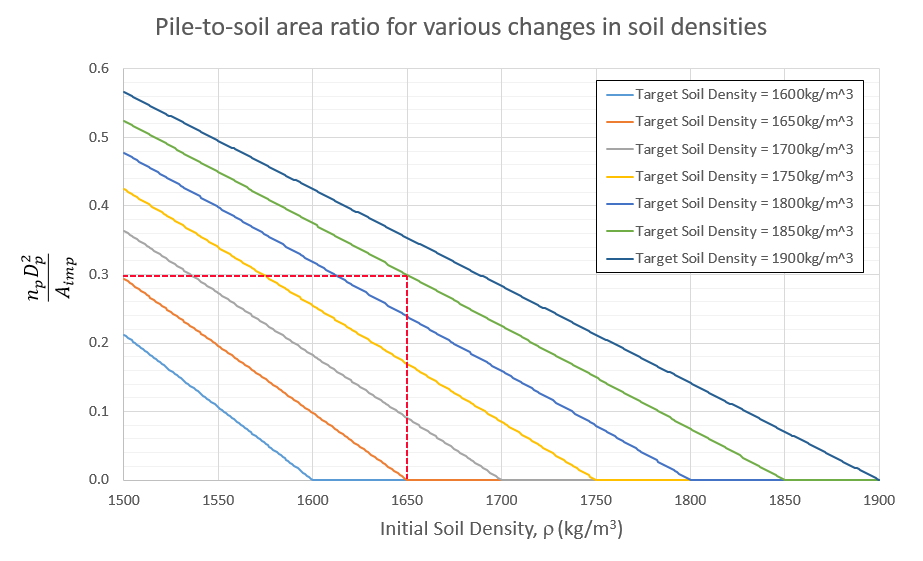Figure 1: Design chart for TCP ground improvement layout based on the change in soil density.

Figure 2 shows a simple design chart that can be used to estimate the total number of piles required for a range of pile diameters, the area of improvement has been assumed to be 300m2. It is estimated that approximately 1000 0.30m diameter piles would need to be installed to achieve the desired increase in soil density; whereas, approximately 440 0.45m diameter piles are required to achieve the same increase.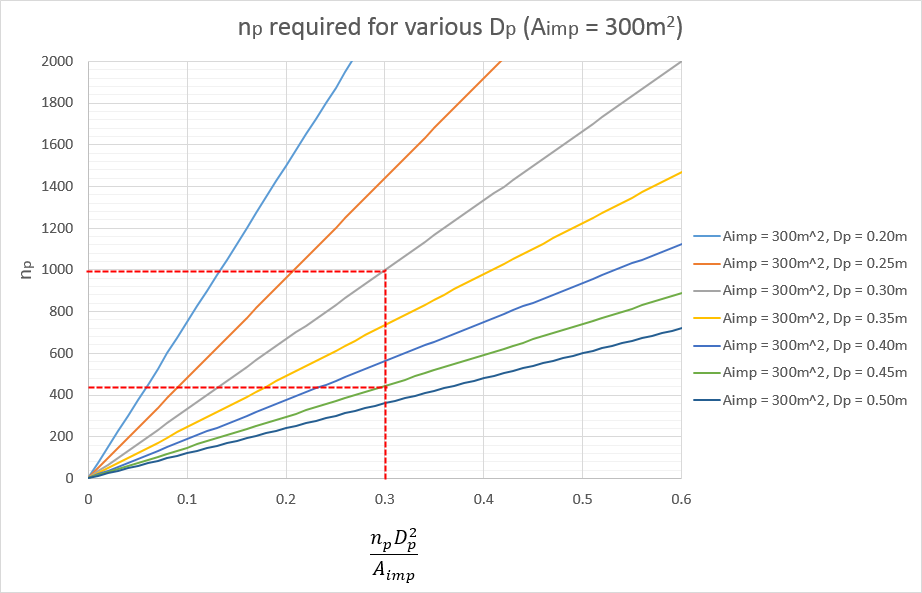Figure 2: Design chart for number of piles required for the given area of improvement for a range of pile diameters.

By following a similar design process, the method can assist at the conceptual design phase to check the feasibility of the ground improvement option, and to select the most cost effective and efficient TCP layout for achieving the target soil density.

# Application of the method to a site in Christchurch

The method has been retrospectively applied to a site in Christchurch, New Zealand where TCP were utilised and both pre-improvement and post-improvement CPTu data is available. The results from the proposed method are compared against the measured change in soil density and stiffness.

## Site characteristics

The site has surficial loose, potentially liquefiable sands and silts overlying denser sands and sandy gravels at approximately 3-4m depth across the site. The site has a shallow groundwater table which was measured at approximately 1.3m depth during the CPTu soundings. The new structure is a relatively long and narrow, lightweight commercial building. As a result, the TCP layout consisted of 21 rows of piles, with six piles in each row across the width of the structure. Key parameters related to the ground improvement works are Aimp = 192m2, np = 126 piles and Dp = 0.35m (i.e. a pile-to-soil area ratio of approximately 0.08).

It should be noted that three of the pre-improvement CPTu soundings (CPT-01 to CPT-03) were carried out prior to the demolition of the original structure (outside the building footprint) and the final pre-improvement CPTu (CPT-04) was carried out close to the centre of the new building footprint just prior to the construction of the TCP. CPT-05 to CPT-09 were carried out within the area of improvement after the installation of the TCP.

Figure 3 shows the indicative pre-improvement SBTn profile and a breakdown of the pre-improvement CPTu data, including the median values for , qc and qc1Ncs to around 5m depth. There is a surficial layer of silty sand and sandy silt overlying a layer of sands and silty sands to approximately 4m depth. The CPT refused in the underlying denser sands and sandy gravels. The soil density is variable across the site; however, below the groundwater table the median soil density gradually increases with depth ranging from 1700-1850kg/m3 in the sand and silty sand layer. The median qc values are approximately 2MPa in the surficial sandy silt layer and 8MPa in the sand and silty sand layer.

 Indicative SBTn Plot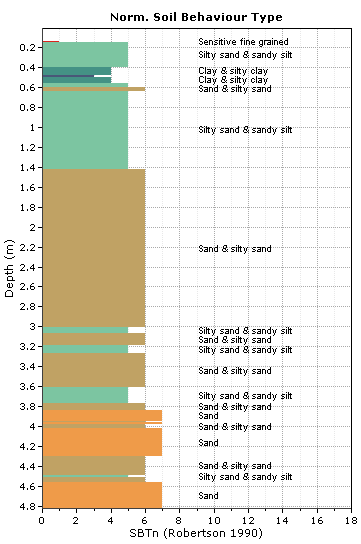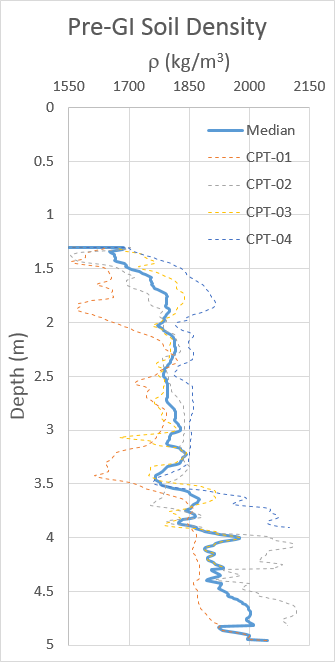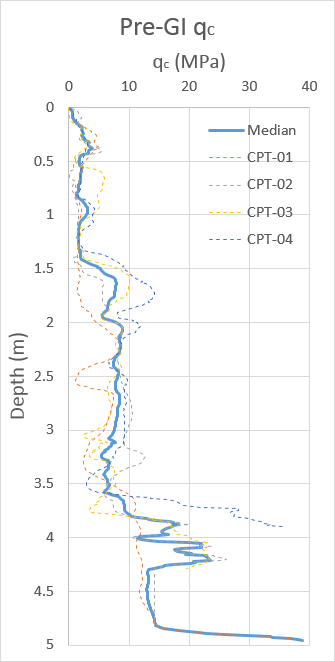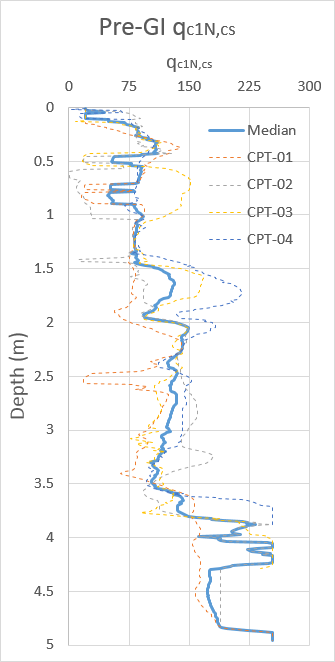Figure 3: Pre-improvement SBTn (left), soil density (centre-left), qc (centre-right), and qc1N, cs (right).

## Post-improvement analysis

The post-improvement CPTu data has been analysed and compared to the pre-improvement CPTu data to determine the changes that have occurred due to the inclusion of TCP. Figure 4 below summarises the median pre-improvement and post-improvement , qc and qc1Ncs values, with e estimated as per Barounis et al. 2017. The soil density consistently increases throughout the soil profile and the void ratio reduces accordingly. The qc and qc1Nc,cs values have increased significantly and exceed the target values included in the MBIE Guidelines throughout the majority of the improved soil layers.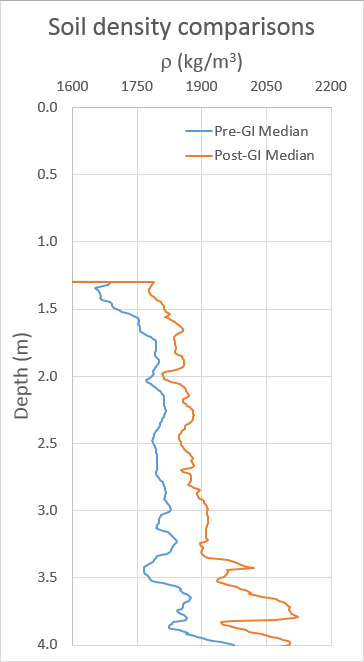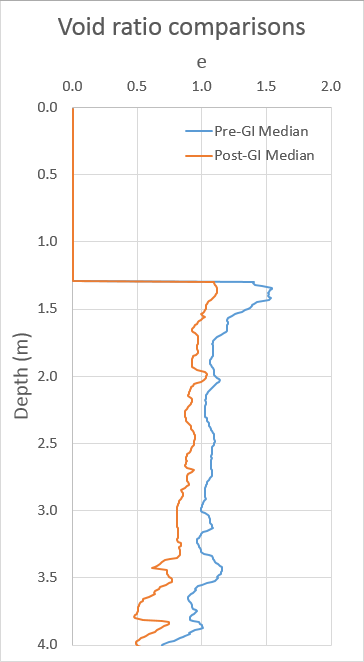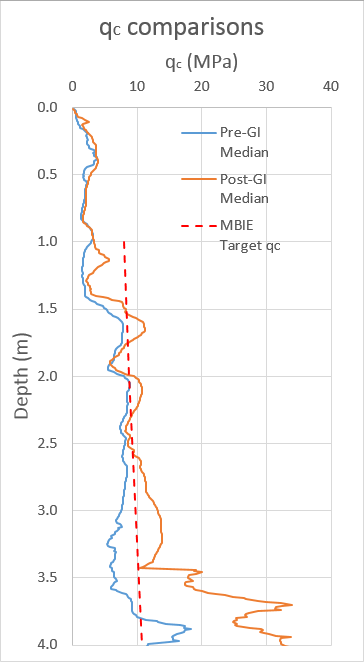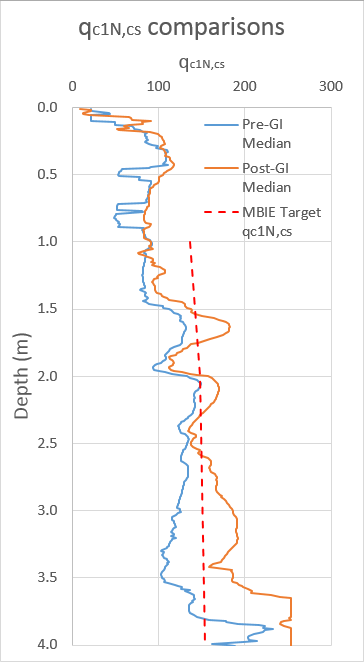Figure 4: Comparisons between median values of pre-improvement and post-improvement soil density (left), void ratio (centre-left), qc (centre-right), and qc1N, cs (right).

Figure 5 shows the absolute change in the median values for ρ, e, qc and qc1NCS due to the inclusion of CTP. The soil density increased by approximately 50-100kg/m3 throughout the sand and silty sand layer. Slightly larger increases in density occurred in the denser sands and sandy gravels at 3.5-4.0m depth. Similarly, the void ratio reduces in the range of 0.1-0.2 throughout the sand and silty sand layer with larger reductions occurring in denser sands and sandy gravels. The increase in the measured qc is in the range of 1-3MPa in the sand and silty sand layer; however, the sandy silts above the groundwater table show a negligible increase in the measured qc. The qc1N,cs values increased by approximately 20-50 in the sand and silty sand layer, and the dense sands and sandy gravels increased in the range of 75-100. Changes in qc1N,cs values were highly variable above the groundwater table.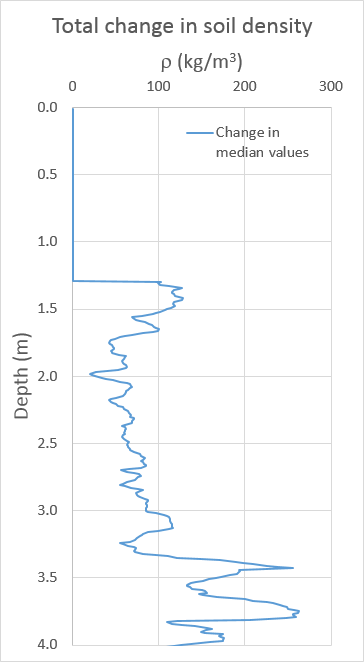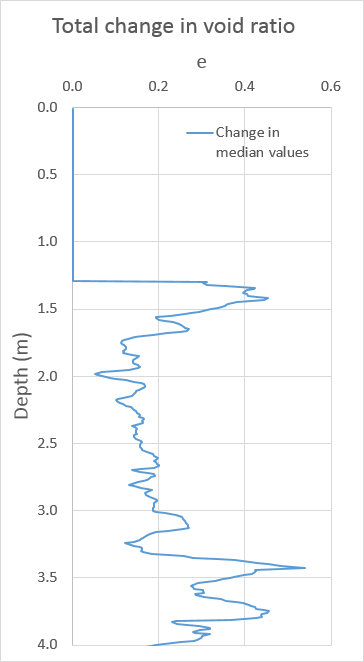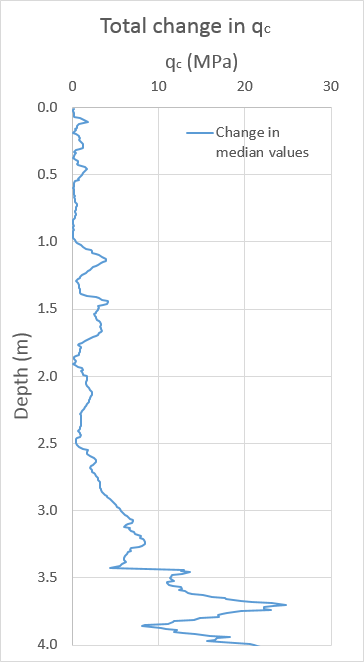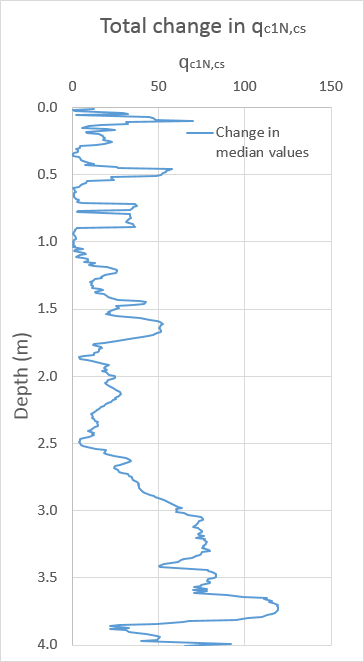Figure 5: Absolute change in the median values of soil density (left), void ratio (centre-left), qc (centre-right), and qc1N, cs (right) due to the installation of TCP.

## Estimating the change in soil density using pre-improvement CPTu data

By applying the proposed method, the post improvement soil density can be estimated from Equation 20 using pre-improvement CPTu data. These results can then be compared to the post-improvement measurements for the given site. Figure 6 shows the measured median soil density, both before and after the TCP inclusion, as well as the estimated soil density from equation 20. The deviation between the measured and estimated soil densities, is also plotted in Figure 6. For the error plot, a positive value indicates the method is underpredicting the change in soil density. The proposed method is producing a reasonable estimate of the post-improvement soil density in the sand and silty sand layer. There are some under predictions occurring at shallow depths and in the denser sands and sandy gravels near the base of the piles.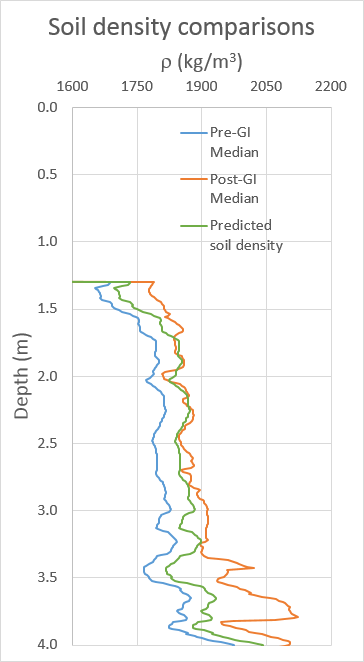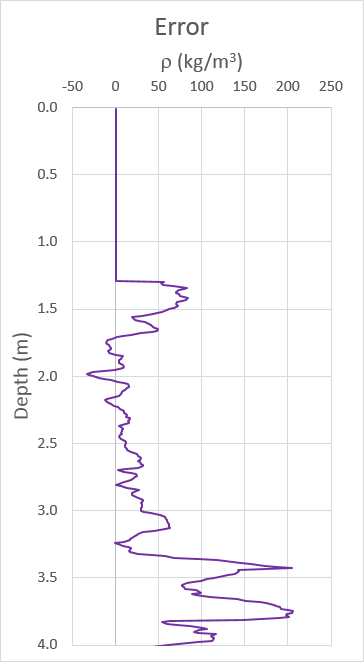Figure 6: Estimated and measured soil densities (left) and the error produced by using the proposed method (right – a positive error means the soil density has been underestimated by the method).

# Discussion and conclusion

The proposed method has been applied to a site in Christchurch with results showing a reasonable estimation of the change in soil density occurring in the saturated sand and silty sand layer. The method is underestimating the degree of improvement achieved above 1.7m as this soil layer may not be completely saturated (Sr < 1.0) and therefore the relationships used may not be reliable in the partially saturated soil. The method appears to be under predicting the density increase in the denser sand and sandy gravel layer. Many of the TCP refused when being driven into the denser gravel layers, which may have resulted in some additional densification of material near the base of the piles.

The method may be useful for assessing the feasibility of using TCP as a ground improvement technique prior to constructing the piles; therefore, reducing the likelihood of the ground improvements not achieving the target density or post-installation stiffness requirements. There are many limitations to the method which need to be taken into consideration when applying the proposed method. More research is recommended to check the validity of the method for a wider range of soil conditions where TCP may be useful to improve the stiffness and density of the soil.

# REFERENCES

Barounis, N. and Philpot, J. 2017. Estimation of in-situ water content, void ratio, dry unit weight and porosity using CPT for saturated sands, Proc. 20th NZGS Geotechnical Symposium.

MBIE. 2012. MBIE Guidance: Repairing and rebuilding houses affected by the Canterbury earthquakes.Barounis_NZGS2021_Submission_Ref-0076_Create (649 KB)